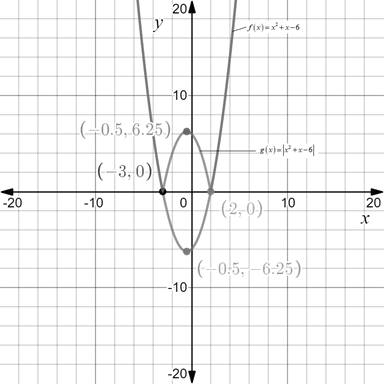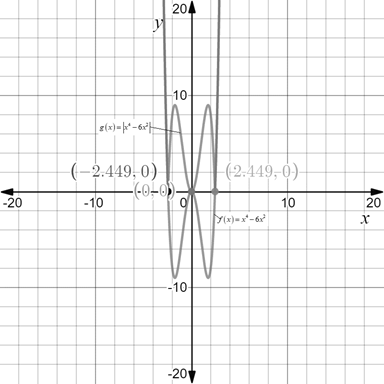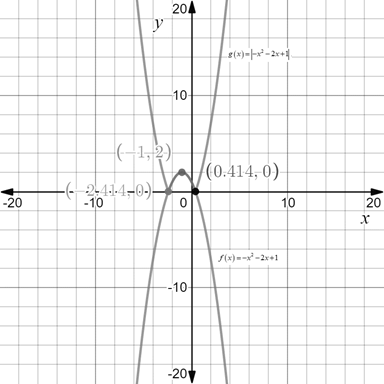# To draw: The graph of the functions f ( x ) = x 2 + x − 6 and g ( x ) = | x 2 + x − 6 | .### Precalculus: Mathematics for Calcu...

6th Edition
Stewart + 5 others
Publisher: Cengage Learning
ISBN: 9780840068071### Precalculus: Mathematics for Calcu...

6th Edition
Stewart + 5 others
Publisher: Cengage Learning
ISBN: 9780840068071

#### Solutions

Chapter 2.2, Problem 87E

(a)

To determine

## To draw: The graph of the functions f(x)=x2+x−6 and g(x)=|x2+x−6| .

Expert Solution

### Explanation of Solution

Given information:

The given functions are f(x)=x2+x6 and g(x)=|x2+x6| .

Graph:

The graph of functions f(x)=x2+x6 and g(x)=|x2+x6| is shown below.Interpretation:

In the graph, the graph for the function g(x)=|x2+x6| opens downward and the graph of the function f(x)=x2+x6 and these intersects each other on the x -axis. Since the graph of g(x) is reflection of the function f(x) above the x -axis. Therefore, the functions are related to each other as follows.

g(x)=|f(x)|

(b)

To determine

Expert Solution

### Explanation of Solution

Given information:

The given functions are f(x)=x46x2 and g(x)=|x46x2| .

Graph:

The graph of functions f(x)=x46x2 and g(x)=|x46x2| is shown below.Interpretation:

In the graph, the graph for the function g(x)=|x46x2| opens downward and the graph of the function f(x)=x46x2 and these intersects each other on the x -axis. Since the graph of g(x) is reflection of the function f(x) above the x -axis. Therefore, the functions are related to each other as follows.

g(x)=|f(x)|

(c)

To determine

### To find: The relation between f and g when g(x)=|f(x)| .

Expert Solution

The function g is reflection of the function f .

### Explanation of Solution

Given information:

The given relation is g(x)=|f(x)| .

Calculation:

Let the function f(x)=x22x+1 and g(x)=|x22x+1| . Draw the graph for these functions.In the graph for the function g(x)=|x22x+1| opens downward and the graph of the function f(x)=x22x+1 and these intersects each other on the x -axis. Since the graph of g(x) is reflection of the function f(x) above the x -axis. Therefore, the functions are related to each other as follows.

Therefore, the function g is reflection of the function f .

### Have a homework question?

Subscribe to bartleby learn! Ask subject matter experts 30 homework questions each month. Plus, you’ll have access to millions of step-by-step textbook answers!